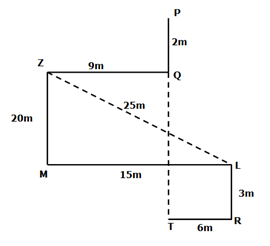# IBPS Clerk Prelims Reasoning Ability Questions 2021 (Day-03)

Dear Aspirants, Our IBPS Guide team is providing new series of Reasoning Questions for IBPS Clerk Prelims 2021 so the aspirants can practice it on a daily basis. These questions are framed by our skilled experts after understanding your needs thoroughly. Aspirants can practice these new series questions daily to familiarize with the exact exam pattern and make your preparation effective.

Start Quiz

Number series

Direction (1-4): Study the following information carefully and answer the below questions

528      439       671        264       863

1) If the numbers are written in descending order within the number from left to right, which of the following number will become the highest number?

A.863

B.264

C.671

D.439

E.528

2) If the second digit of each number is dropped, then the first and last digits of each number are interchanged, which of the following will be the lowest number?

A.863

B.528

C.671

D.439

E.264

3) If the position of the second and last digits are interchanged then how many numbers will be divisible by two?

A.1

B.2

C.3

D.4

E.none

4) What is the sum of the second digit ofthe highest number and the third digit of the lowest number?

A.10

B.14

C.12

D.8

E.6

Order and ranking

5) In a class, Raju is ranked 18th from the bottom and Mahesh is ranked 15th from the top. 8 persons have failed in at least one subject in the examination. If Raju is ranked immediately after Mahesh then how many students are in the class?

A.41

B.33

C.35

D.40

E.None of the above

Direction sense

Direction (6-8): Study the following information carefully and answer the below questions

Point P is 2m north of Point Q. Point Z is 9m west of Point Q. Point L is 15m east of Point M which is 20m south of Point Z. Point R is 3m south of Point L. Point T is west of point R and South of Point P.

6) How far and what is the direction of Q with respect to T?

A.21m towards the south

B.20m towards the south

C.23m towards the north

D.22m towards the north

E.None of the above

7) What is the shortest distance between Point L and Point Z?

A.21m

B.24m

C.20m

D.23m

E.25m

8) What is the shortest distance between T and R?

A.6m

B.15m

C.7m

D.9m

E.None of the above

Inequality

Direction (9-10): In these questions, the relationship between different elements is shown in the statements. These statements are followed by two conclusions. Find the conclusion which logically follows.

(a) If only conclusion I follow.

(b) If only conclusion II follows.

(c) If either conclusion I or II follows.

(d) If neither conclusion I nor II follows.

(e) If both conclusion I and II follow.

9) Statements:

P ≥ N = G > T < K > L

Conclusion:

I). G < K

II). P > T

10) Statements:

Q > K > L ≤ C = H > I

Conclusion:

I). K > I

II). H > L

Given series

528 439 671 264 863

After arranged in descending order from left to right

852  943 761 642 863

Given series

528 439 671 264 863

After the second digit dropped

58 49 61 24 83

After the First and last digits interchanged

85 94 16 42 38

Given series

528 439 671 264 863

Second and Last digits interchanged

582 493 617 246 836

Given series

528 439 671 264 863

6+4=10

18+15+8 = 41

Directions (6-8) :202+152=ZL2

√(202+152)=√625=25m